Next: 5.1.2 Modeling of Conventional Up: 5.1 Exposure Kinetics Previous: 5.1 Exposure Kinetics

## 5.1.1 Absorption in a Dilute Solution

The basic law of light absorption in matter is an empirical one. It postulates that the decrease of the light intensity is proportional to the intensity itself. For a wave traveling in vertical z-direction we thus write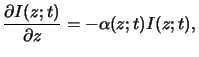(5.1)

whereby the generally spatial- as well as time-dependent quantity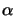(z;t) is the absorption coefficient of the medium. This relation is commonly called Lambert's law [11, p. 614] and has the interpretation of a probability law, i.e., the probability that a photon will be absorbed is proportional to the photon flux. Simple integration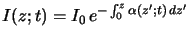(5.2)

yields the absorbance of the medium that is defined as the exponential term in the above equation. The absorbance is the second important characteristic quantity of the absorption phenomenon and often used as an integral measure of the photoresist.

In case of a time-harmonic EM field conveniently described by a phasor notation (cf. (4.1)), the absorption coefficient(x;t) can be related to the imaginary part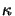of the complex-valued index of refraction n bya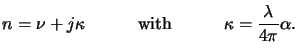(5.3)

The quantityis commonly called the extinction coefficient of the medium. By using the complex-valued index of refraction thus defined the light absorption inside the medium while propagating through it is implicitly accounted for. The above relation (5.3) provides therefore an elegant way of describing both phenomena of field propagation and absorption.

In case of a dilute solution the absorption coefficient(x;t) introduced in Lambert's law (5.1) is proportional to the concentration C(x;t) of the absorbing species, i.e.,(5.4)

which is commonly referred to as Beer's law . This simple relation starts to fail at high particle concentration as it is based on the assumption of complete independence of the absorbing molecules. If the particles get too close to each other they mutually influence each other. Since any interaction depends on the concentration C(x;t), the linear relation postulated by Beer's law in (5.4) breaks down. Another apparent deviation from (5.4) occurs if the real part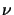of the refraction index n strongly depends on the concentration. Thus the validity of Beer's law is not always guaranteed but should be checked over the concentration range of interest.

A simple extension of (5.4) applies to materials consisting of multiple absorbing components. In such a situation the overall absorption coefficient can be written as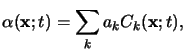(5.5)

provided that the independence also holds across the different absorbing species. The basic results stated in (5.1) to (5.5) will now be used for the modeling of the two resist systems of interest.

#### Footnotes

... bya
The spatial- and time-dependence is suppressed for the moment.Next: 5.1.2 Modeling of Conventional Up: 5.1 Exposure Kinetics Previous: 5.1 Exposure Kinetics
Heinrich Kirchauer, Institute for Microelectronics, TU Vienna
1998-04-17